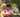### PermCheck

February 07, 2020

PermCheck

A non-empty array A consisting of N integers is given.
A permutation is a sequence containing each element from 1 to N once, and only once.

For example, array A such that:
A = 4
A = 1
A = 3
A = 2
is a permutation, but array A such that:
A = 4
A = 1
A = 3
is not a permutation, because value 2 is missing. The goal is to check whether array A is a permutation.

Write a function:
function solution(A);
that, given an array A, returns 1 if array A is a permutation and 0 if it is not.
For example, given array A such that:
A = 4
A = 1
A = 3
A = 2
the function should return 1.

Given array A such that:
A = 4
A = 1
A = 3
the function should return 0.

Write an efficient algorithm for the following assumptions:
• N is an integer within the range [1..100,000];
• each element of array A is an integer within the range [1..1,000,000,000].

``````function solution(A) {
// write your code in JavaScript (Node.js 8.9.4)

A.sort((a,b)=>a-b)
if(A !== 1) return 0

for(let i=1; i<A.length; i++){
if( A[i] !== A[i-1]+1) return 0
}
return 1

}``````Posted by Tai Lu ( 呂台生 ) who's building useful things.You should follow him on Twitter